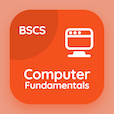Engineering Online Courses

Engineering Mathematics MCQs

# Engineering Math Exam Quizzes, Trivia, Questions and Answers MCQ with Answers PDF

Engineering Math Exam Quizzes, Trivia, Questions and Answers Multiple Choice Questions (MCQ), Engineering Mathematics quiz answers PDF to study engineering mathematics online course for engineering mathematics classes. Complex Numbers and Functions Multiple Choice Questions and Answers (MCQs), Engineering Mathematics quiz questions for engineering graduate colleges. "Engineering Math Exam Quizzes, Trivia, Questions and Answers " PDF Book: differential equations final exam questions with solutions, advanced maths quiz questions with answers, differential equations questions with solutions, differential equations final exam with solutions test prep for job assessment test.

"Complex line integral is taken over" MCQ PDF: engineering math exam quizzes, trivia, questions and answers with choices infinity, randomly, fixed path, and exponent for engineering graduate colleges. Study engineering math exam quizzes, trivia, questions and answers quiz questions for merit scholarship test and certificate programs for job assessment test.

## MCQs on Engineering Math Exam Quizzes, Trivia, Questions and Answers Quiz

MCQ: Complex line integral is taken over

infinity
randomly
fixed path
exponent

MCQ: A sequence in Taylor series obtained by assigning to each positive integer n a number, called sequence

unit
term
core
path

MCQ: Every power series converges at the

left side
right side
center point
initial point

MCQ: A representation of a function as an infinite sum of terms that are calculated from the values of the function' derivatives at a single point is called

Taylor series
Fourier series
Laplace transforms
integration

MCQ: A real sequence is one whose terms are

imaginary
real
characters
string

### More Topics from Engineering Mathematics Course